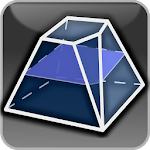500K+

# Geometryx: Geometry - Calculator APK

Version: Varies with device code 23

Developer: famobix

Released: 2017-05-20

Updated: 2020-11-01## Description of Geometryx: Geometry - Calculator APK

Geometryx is an application that allows you to calculate quickly and easily the most important values and parameters of plane and solid figures and shapes.
The application calculates an area, perimeter, circumference, diagonal length, volume, coordinates of the geometric centroid, height, side lenght, angle (acute, right, obtuse, straight, reflex), radius (inner, outer), edges, arc length, line segments, base area, lateral surface area and total surface area of three-dimensional geometric shapes.
Geometryx is a simple calculator using trigonometric functions, Pythagorean theorem and Thales' theorem.
The application calculates many geometric figures, among others triangle, square or circle and even more solids i.e. cube, cone and sphere.

Geometryx also includes the most significant geometric formulas and equations that help you solve any problems and tasks in geometry.
Thanks to this application, the geometry will become very simple. Geometryx will be helpful for students, teachers, scientists, engineers, technicians, and anyone who has contact with geometry.

Geometryx = Great Geometry EXperience !

List of plane and solid figures contained in the application:
Planimetry ( 2D Geometry ) and Stereometry ( 3D Geometry )

Update on
2020-11-01
Package name
com.famobix.geometryx
Author
famobix
Version name
Varies with device
Installs
500.000++
File size
8.307.334 bytes
Category
EDUCATION
Requirements
Android Varies with device+
Content rating
Rated for 3+